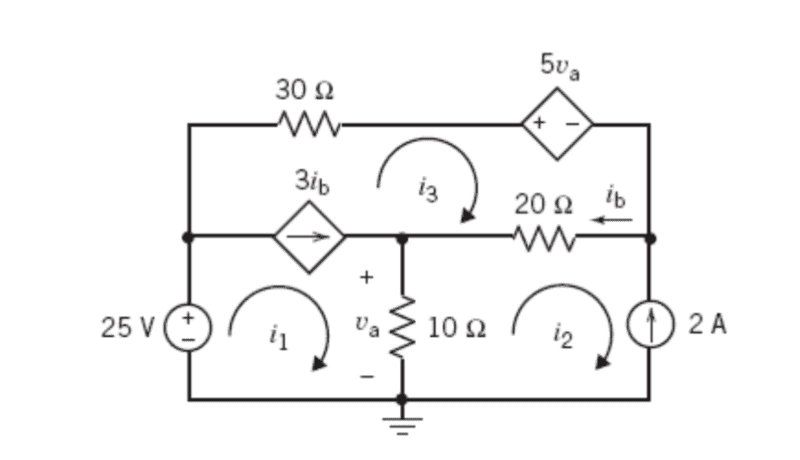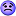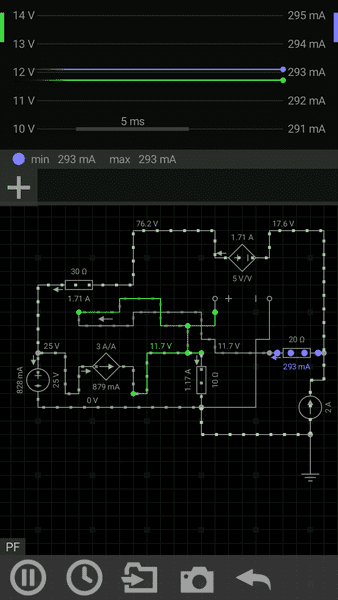# Arbitrary current with mesh analysis

Members are reminded to use the 3 header template in the homework help forums
Given the circuit in the diagram I was wondering would I be able to make ib equal to the mesh i3.I have the equation for my Super Mesh at i1 and i3 which is:

-25+30i3+5Va+20(i3-i2)+10(i1-i2)=0 (1).

The mesh i2 is equal to -2A (2).

subbing (2) into (1) gives:
-25+50i3+5Va-30i2+10i1=0 (3).

Va=i1-i2 (4).

subbing (4) into (3) gives: -25+50i3-35i2+15i1=0 (5).

subbing (2) into (5).
45+50i3+15i1=0 (6).

3ib=i1-i3 (7).

Now I almost have one unknown. Is it possible to set ib equal to i3 so i can isolate for i1 in equation 7. If i can't how would i be able to solve it.

Also, are the steps to finding the mesh currents correct for this problem?

NascentOxygen
Staff Emeritus
ib is the current through the 20Ω (from right to left). I can see that resistor's current is composed of 2 mesh currents, I3 flowing R to L, and I2 flowing L to R.

Your eqn (4) is missing a 10, it's a 10Ω resistor.

The current through the 10Ω can also be equated to the sum of the currents into the node above it.

•scottdave
ib is the current through the 20Ω (from right to left). I can see that resistor's current is composed of 2 mesh currents, I3 flowing R to L, and I2 flowing L to R.

Your eqn (4) is missing a 10, it's a 10Ω resistor.

The current through the 10Ω can also be equated to the sum of the currents into the node above it.
What do you mean the current of the 10Ω resistor? Do you mean mesh current 3?

NascentOxygen
Staff Emeritus
What do you mean the current of the 10Ω resistor? Do you mean mesh current 3?
I am speaking of the two currents 3ib and ib.

What it is still missing is: i1=3*ib-i3 and since ib=i3+2 and ua=10*(i1-i2) you may reduce the first equation so that only i3 stays as unknown.

•scottdave
What it is still missing is: i1=3*ib-i3 and since ib=i3+2 and ua=10*(i1-i2) you may reduce the first equation so that only i3 stays as unknown.
After doing all of the calculations is an answer for mesh current 1 -17/27A correct?

gneill
Mentor
After doing all of the calculations is an answer for mesh current 1 -17/27A correct?
Nope.

The Electrician
Gold Member
After doing all of the calculations is an answer for mesh current 1 -17/27A correct?

I have the equation for my Super Mesh at i1 and i3 which is:

-25+30i3+5Va+20(i3-i2)+10(i1-i2)=0 (1).

The mesh i2 is equal to -2A (2).

subbing (2) into (1) gives:
35+50i3+5Va+10i1=0 (3).

Va=10(i1+2) (4).
Va=10i1+20 (4)

subbing (4) into (3) gives: 135+50i3+60i1=0 (5).

3ib=i1-i3 (6).

ib=i3-i2 (7)

subbing (7) into (6)
3i3-3i2=i1-i3
i1=4i3-3i2
i1=4i3+6 (8)

subbing (8) into (5)
135+50i3+240i3+360
290i3=-495
i3=-99/58

I was wondering if i went wrong anywhere in my steps?

Last edited:
The Electrician
Gold Member
I have the equation for my Super Mesh at i1 and i3 which is:

-25+30i3+5Va+20(i3-i2)+10(i1-i2)=0 (1).

The mesh i2 is equal to -2A (2).

subbing (2) into (1) gives:
35+50i3+5Va+10i1=0 (3).

Va=10(i1+2) (4).
Va=10i1+20 (4)

subbing (4) into (3) gives: 135+50i3+60i1=0 (5).

3ib=i1-i3 (6).

ib=i3-i2 (7)

subbing (7) into (6)
3i3-3i2=i1-i3
i1=4i3-3i2
i1=4i3+6 (8)

subbing (8) into (5)
135+50i3+240i3+360
290i3=-495
i3=-99/58

I was wondering if i went wrong anywhere in my steps?

You made a sign error with respect to i2 in the red part above. Fix this and recalculate.

You made a sign error with respect to i2 in the red part above. Fix this and recalculate.

Well 20(-1)(-2)=40 and 10(-1)(-2)=20

So -25+40+20=35

where is the sign error

The Electrician
Gold Member
Well 20(-1)(-2)=40 and 10(-1)(-2)=20

So -25+40+20=35

where is the sign error

Never mind. I had a situation of poor visibility on my laptop, and the 35 looked like 85.

You're all good. i3 does indeed equal -99/58

NascentOxygen
Staff Emeritus
I was wondering if i went wrong anywhere in my steps?
The customary check is to substitute your provisional 3 answers back into the 3 equations. If all equations are satisfied, then conclude you have the correct solution to those equations. That works out faster than waiting for someone to look over your calculations....and running the risk of having them miss something anyway.The Electrician
Gold Member
running the risk of having them miss something anyway.Your above eq.no.6 it is incorrect.
It has to be i1=3ib-i3.
Then i3= -2.9117647 A.

NascentOxygen
Staff Emeritus
Your above eq.no.6 it is incorrect.
It has to be i1=3ib-i3.
Then i3= -2.9117647 A.
OP's eqn 6 is correct. You were wrong in post #5

It may be but this is the solution. Check this with first equation and see it fits.
i3=-2.9117647 A
i2=-2
ib=i3-i2=-0.9117647
i1=3*ib-i3=3*(-0.9117647)+2.9117647=0.1764706 A
ua=10*(i1-i2)=10*(2.1764706)=21.764706 V
SUM=-25+30*i3+5*ua+20ib+ua=0

The Electrician
Gold Member
I get:
i1 = -.82759
i2 = -2
i3 = -1.7069

Check -25+30*i3+5*ua+20*ib+ua=204.827 with your results, but has to be 0.

The Electrician
Gold Member
Check -25+30*i3+5*ua+20*ib+ua=204.827 with your results, but has to be 0.

OK, let's evaluate all the terms using my values for i1, i2 and i3, using the fact that ua = 10*(i1-i2), ib = i3-i2
Listing the results, I get:
-25 = -25
30*i3 = -51.2068965516
5*ua = 58.620689655
20*ib = 5.8620689656
ua = 11.724137931

Adding all these up, I get zero, not 204.827

NascentOxygen
Staff Emeritus
Perhaps @garr6120 has been given the solution?

It is very interesting: both results seem to be correct.

cnh1995
Homework Helper
Gold Member
It is very interesting: both results seem to be correct.
I ran it through a simulation, and the results agree with @The Electrician's solution.OK, let's evaluate all the terms using my values for i1, i2 and i3, using the fact that ua = 10*(i1-i2), ib = i3-i2
Listing the results, I get:
-25 = -25
30*i3 = -51.2068965516
5*ua = 58.620689655
20*ib = 5.8620689656
ua = 11.724137931

Adding all these up, I get zero, not 204.827

The Electrician
Gold Member
It is very interesting: both results seem to be correct.

Check -25+30*i3+5*ua+20*ib+ua, has to be 0.

OK, let's evaluate all the terms using your values for i1, i2 and i3, using the fact that ua = 10*(i1-i2), ib = i3-i2
Listing the results, I get:

-25 = -25
30*i3 = 87.352941
5*ua = 108.82353
20*ib = 98.235294
ua = 21.764706

These add up to 291.176471, not zero

Something goes wrong in your last calculation The Electrician. See post no.17 for i3 and ib values.